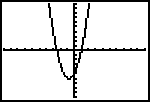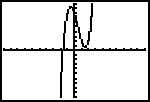Go to content

Investigate the effect of changing $a$ and $b$.

Make sure that you are clear about these aspects of a quadratic graph:

• its basic shape
• its symmetry
• the number of places where the curve crosses the x-axis
• how the values of $a$ and $b$ affect the shape and position of the curve

Summary/BackgroundA graphic calculator is not allowed in your exam. However you can use one for revision.

The TI-83, or similar model, can display cubic graphs for you:

• Select the $Y=$ screen
• Enter $Y1 = AX$x2$+BX+C$
• Then select the GRAPH screen.
• You can then choose different values for the constants.
• For example, to make $A$ = $1$ press $1$ STO➧ ALPHA $A$.

JSXGraph is a cross-browser library for interactive geometry, function plotting, charting, and data visualization in a web browser. It is implemented completely in JavaScript, does not rely on any other library. It uses SVG and VML and is fully HTML5 compliant.

Glossary

cubic

A mathematical expression of the 3rd degree; having a x³ term but no higher one.

function

A rule that connects one value in one set with one and only one value in another set.

graph

A diagram showing a relationship between two variables.
The diagram shows a vertical y axis and a horizontal x axis.

A mathematical expression of the 2nd degree; having a x² term but no higher one.

Full Glossary List

This question appears in the following syllabi:

SyllabusModuleSectionTopic
AP Calculus AB (USA)2Algebra and FunctionsQuadratic curves
AP Calculus BC (USA)2Algebra and FunctionsQuadratic curves
AQA A-Level (UK - Pre-2017)C1Algebra and FunctionsQuadratic curves
AQA AS Maths 2017Pure MathsAlgebraQuadratic Curves
AQA AS/A2 Maths 2017Pure MathsAlgebraQuadratic Curves
CBSE IX (India)AlgebraPolynomialsConstant, linear, quadratic and cubic
CCEA A-Level (NI)C1Algebra and FunctionsQuadratic curves
CIE A-Level (UK)P1Algebra and FunctionsQuadratic curves
Edexcel A-Level (UK - Pre-2017)C1Algebra and FunctionsQuadratic curves